Open In App
Related Articles
• RD Sharma Class 12 Solutions for Maths

# Class 12 RD Sharma Solutions – Chapter 23 Algebra of Vectors – Exercise 23.4

###Solution: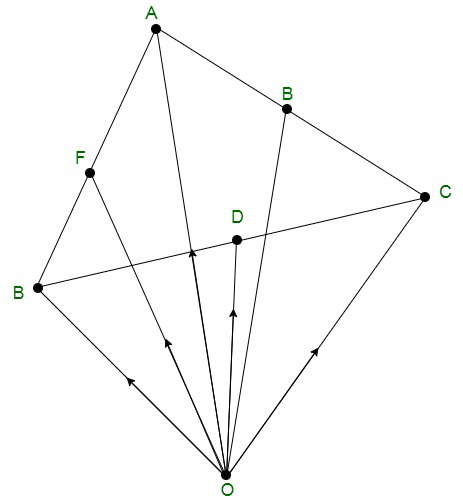In the △ABC, D, E, F are the mid points of the sides of BC, CA and AB and O is any point in space.

Let us consideredbe the position vector of point A, B, C, D, E, F with respect to O.

Therefore,So, according to the mid-point formulaHence, proved### Question 2. Show that the sum of three vectors determined by the medians of a triangle directed from the vertices is zero.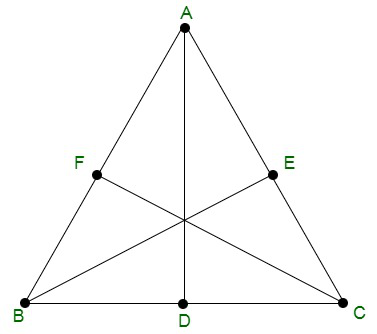Solution:

Let us considered ABC is triangle, so the position vector of A, B and C areHence, AD, BE, CF are medium, so, D, E and F are mid points of line BC, AC, and AB.

Now, using mid point formula we get

Position vector of D =Position vector of E =Position vector of F =Now, add all the three medianHence, proved that the sum of the three vectors determined by the medians

of a triangle directed from the vertices is zero.

### Question 3. ABCD is a parallelogram and P is the point of intersection of its diagonals. If O is the origin of reference, show that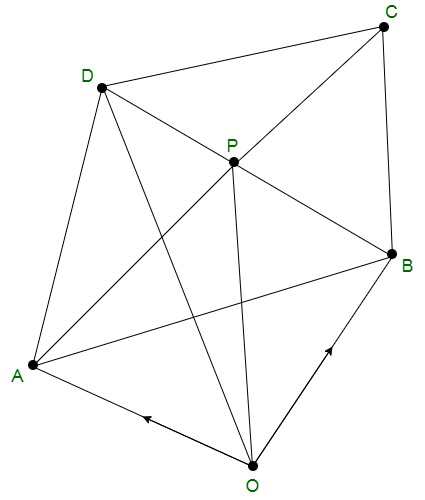Solution:

Given that ABCD is a parallelogram, P is the point of intersection of diagonals and O be the point of reference.

So, by using triangle law in △AOP, we get-(1)

By using triangle law in △OBP, we get-(2)

By using triangle law in △OPC, we get-(3)

By using triangle law in △OPD, we get-(4)

Now, on adding equation (1), (2), (3) and (4), we get### Question 4. Show that the line segments joining the mid-points of opposite sides of a quadrilateral bisect each other.

Solution: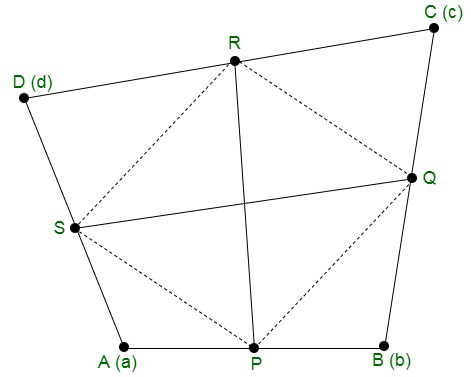Let us considered ABCD be a quadrilateral and P, Q, R, S be the mid points of sides AB, BC, CD and DA.

So, the position vector of A, B, C and D beUsing the mid point formula

Position vector of P =Position vector of Q =Position vector of R =Position vector of S =Position vector of= Position vector of Q – Position vector of P-(1)

Position vector of= Position vector of R – Position vector of S-(2)

From eq(1) and (2),So, PQRS is a parallelogram and PR bisects QS             -(as diagonals of parallelogram)

Hence, proved that the line segments joining the mid-points of

opposite sides of a quadrilateral bisect each other.

### Question 5. ABCD are four points in a plane and Q is the point of intersection of the lines joining the mid-points of AB and CD; BC and AD. Show thatwhere P is any point.

Solution: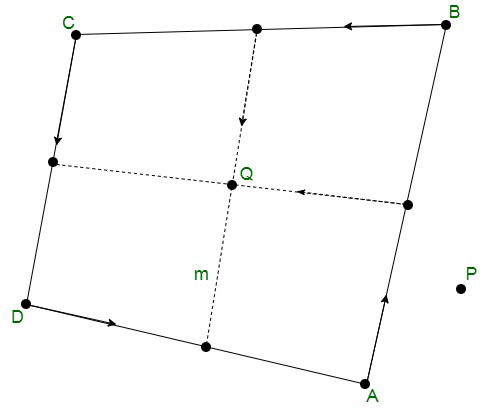Let us considered the position vector of the points A, B, C and D areUsing the mid-point formula, we get

Position vector of AB =Position vector of BC =Position vector of CD =Position vector of DA =It is given that Q is the mid point of the line joining the mid points of AB and CD, soNow, let us assumebe the position vector of P.

So,Hence proved

### Question 6. Prove by vector method that the internal bisectors of the angles of a triangle are concurrent.

Solution: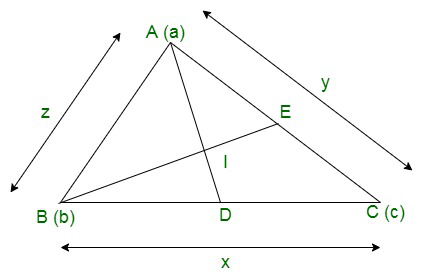In triangle ABC, let us assume that the position vectors of the vertices of the triangle areLength of the sides:

BC = x

AC = y

AB = z

In triangle ABC, the internal bisector divides the opposite side in the ratio of the sides containing the angles.

Since AD is the internal bisector of the ∠ABC, so

BD/DC = AB/AC = z/y          -(1)

Therefore, position vector of D =Let the internal bisector intersect at point I.

ID/AI = BD/AB         -(2)

BD/DC = z/y

Therefore,

CD/BD = z/y

(CD + BD)/BD = (y + z)/z

BC/BD = (y + z)/z

BD = ln/y + z         -(3)

So, from eq(2) and (3), we get

ID/AI = ln/(y +z)

Therefore,

Position vector of I =Similarly, we can also prove that I lie on the internal bisectors of ∠B and ∠C.

Hence, the bisectors are concurrent.